Next: General comments Up: Examples and Troubleshooting Previous: Example 3   Contents

Example 4

In this example (see section 15.1.3) we deal with a complex problem of three equations in three unknowns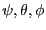. We are looking for a solution in the domain: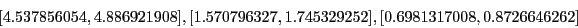The system has a solution which is approximately: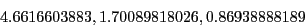This problem is extremely ill conditioned as for the TestDomain the functions intervals are: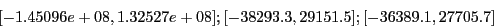This program is implemented under the name Test_Solve_General. With espsilonf=0 and epsilon=0.001 and if we stop at the first solution we find with the maximum equation ordering: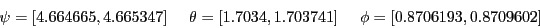with 531 boxes. We may also mention the following remarks:
• we get no improvement with the single bisection mode as we need 2435 boxes to find the first solution,
• using the Reverse Storage mode does not lead to any improvement for finding the first root: in this mode we need 5587 boxes to get the first solution,
With the maximum middle-point equation ordering we find: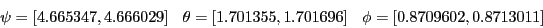with 203 boxes. The importance of normalizing the functions appears if we use epsilonf=0.1 and epsilon=0. If we stop at the first solution we find: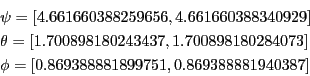while if we divide the first function by 1000 we find: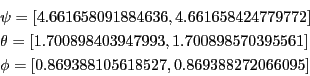in four time less computation time.Next: General comments Up: Examples and Troubleshooting Previous: Example 3   Contents
Jean-Pierre Merlet 2012-12-20